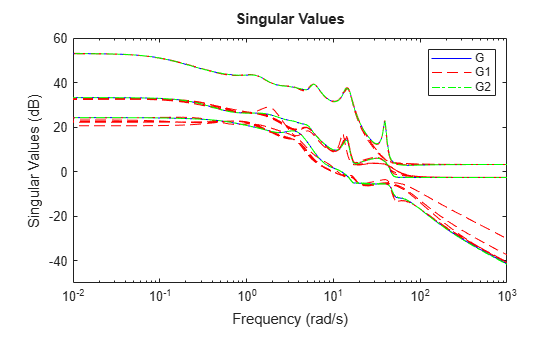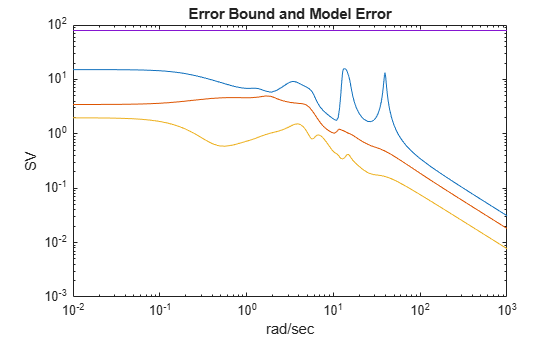# Approximate Plant Model by Additive Error Methods

Given a system `G` in LTI form, the following commands reduce the system to any desired order you specify. The judgment call is based on its Hankel singular values.

```rng(1234,'twister'); G = rss(30,4,3); % random 30-state model % balanced truncation to models with sizes 12:16 [G1,info1] = balancmr(G,12:16); % Schur balanced truncation by specifying `MaxError' [G2,info2] = schurmr(G,'MaxError',[1,0.8,0.5,0.2]); sigma(G,'b-',G1,'r--',G2,'g-.') legend('G','G1','G2')```The plot compares the original model `G` with the reduced models `G1` and `G2`.

To determine whether the theoretical error bound is satisfied, calculate the peak difference across frequencies between the gain of the original system and the reduced system. Compare that to the error bound stored in the `info` structure.

`norm(G-G1(:,:,1),'inf') `
```ans = 2.2965 ```
`info1.ErrorBound(1)`
```ans = 9.7120 ```

Or, plot the model error vs. error bound via the following commands:

```[sv,w] = sigma(G-G1(:,:,1)); loglog(w,sv,w,info1.ErrorBound(1)*ones(size(w))) xlabel('rad/sec');ylabel('SV'); title('Error Bound and Model Error')```